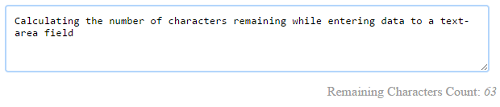# Twitter Style Remaining Character Count using jQuery

In this tutorial, we will learn how to calculate the remaining character count using jQuery. By calculating this count, we can restrict the maximum length of data to be entered by the user.

In the previous tutorial, we have seen restricting the user from entering more than 1 URL into an input field.

In this example, we call a jQuery function on the key-up event of the form input. This function will calculate the remaining character count from the max-length given and the number of characters entered already.

View Demo

## HTML Text-Area to calculate Character Length

This HTML code contains a text area and a button to trigger the jQuery function to calculate the remaining character count.``````<textarea id="text-content" cols="80" rows="4" onKeyup="count_remaining_character()"></textarea>
<div id="character-count" align="right">150</div>
``````

## jQuery Function Calculating Remaining Character Count

This function calculates the number of characters entered by the user. From this number, it calculates the remaining character count and updates it for the user. If the max length exceeds, it will mark the negative number in red.

``````function count_remaining_character() {
var max_length = 150;
var character_entered = \$('#text-content').val().length;
var character_remaining = max_length - character_entered;
\$('#character-count').html(character_remaining);
if(max_length < character_entered) {
\$('#character-count').css('color','#FF0000');
} else {
\$('#character-count').css('color','#A0A0A0');
}
}
``````